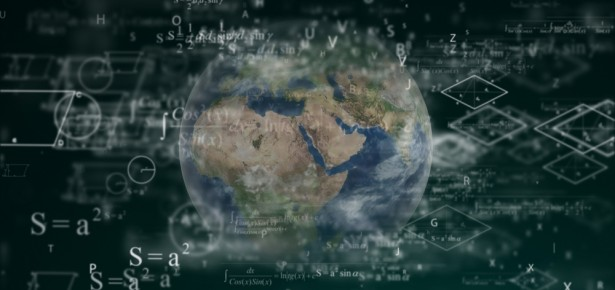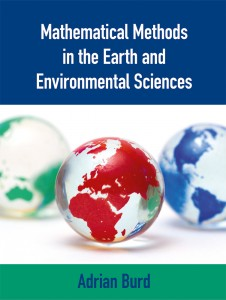Products and services

# Fifteen Eighty Four

26
Apr
2019

## Making mathematical methods less intimidatingMy research career has taken a somewhat non-linear path, starting in theoretical cosmology and ending up in marine sciences. Each transition has required me to learn new things, and so many years ago I was involved in an Antarctic Field Training Course run by the US National Science Foundation. The course was highly interdisciplinary, and as a modeler, I was there to learn about field methods, though the course did include the use of a computer model to help synthesize some of the field data participants would collect. Two things struck me. The first was that other scientists would approach me to help solve a mathematical problem essential to their project for the course. The second was that many participants viewed the use of the model with some degree of trepidation, but once they became comfortable with the model, were excited to see how it helped them explain their data and put it into a wider context.

Today’s students in Earth and environmental sciences face a transition with the increased use of new techniques and computer models to analyze, synthesize, and understand large spatial and temporal data sets. To use these models and techniques effectively requires at least a passing acquaintance with the mathematical concepts and methods that underlie them. However, many students are either intimidated by the subject or have not used the mathematics they know for many years and so find themselves in need of a gentle reminder.

I wanted to write a textbook that presents an unintimidating introduction to the basic mathematical techniques that students will likely encounter. The material in the book is based on three courses that I teach at the undergraduate and graduate levels, and which cover quantitative methods and basic oceanographic and climate modeling. These courses are designed with many opportunities for students to develop and test their understanding by working through problems.  These vary from those that fill in the steps of a worked example to more complex problems requiring the students to formulate equations and develop an appropriate path to their solution. Although I teach mostly students from marine sciences, students from other disciplines such as atmospheric sciences, ecology, geology, and even economics have taken these courses. This has led me to develop examples and assignment problems that cover a range of disciplines in the Earth and environmental sciences, and many of these have found their way into the book.

A computer is now an essential scientific tool. Many mathematical methods have been implemented in a wide variety of programming languages and a series of Matlab and Python codes illustrating some of these have been written to supplement the material in the book.

I hope that this book gives the students and researchers the mathematical tools they need to better understand complicated data analysis techniques and the inner workings of computer models.Mathematical Methods in the Earth and Environmental Sciences
by Adrian Burd, University of Georgia